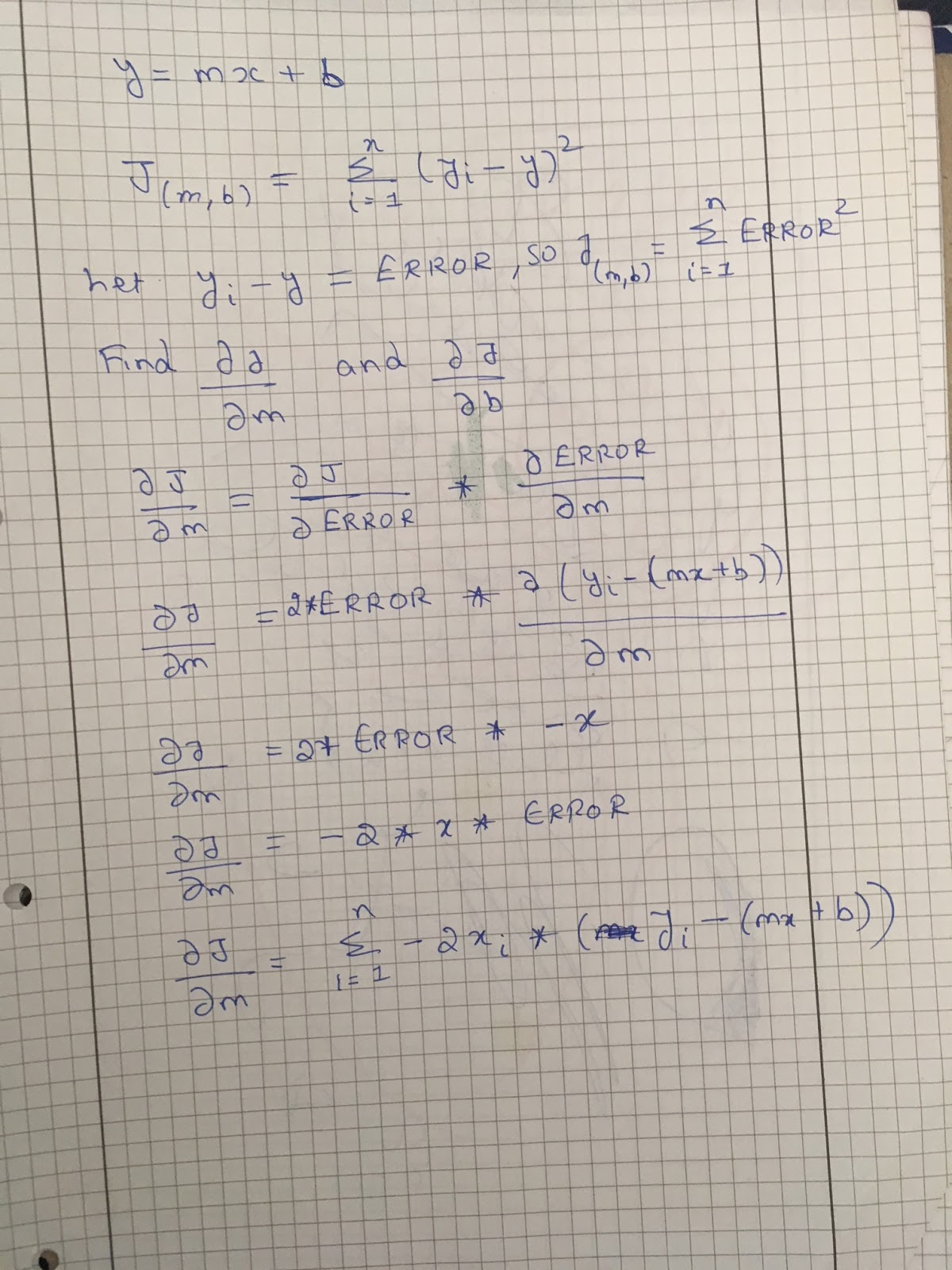# Math behind simple Linear Regression

Entry ticket into the world of ML

joesan published on

2 min, 249 words

Tags: blog

I have been wondering on how the math behind a Linear regression works as in most of the ML books that you encounter, the focus will be on giving you a linear equation and just plugging this equation in a Python library to solve for the slope and bias and then use it to predict the new values. It is very rare that they show you how to find the m and b values. So here in a piece of paper, I decided to try that out, it worked out very well! So if you want to learn it, try to understand what partial derivatives are!In the above solution, I have just solved for m, which is the slope term in a Linear Regression. You can apply the same technique to solve for b! So what you effectively do is to differentiate one term while treating the others as a constant. In simple terms, this is called a partial derivative. A derivative is a measure of something that changes while a partial derivative is a measure of something that changes while treating everything else in this world as a constant! It's that simple! Have a look here at the Wikipedia article on Partial Derivatives!

What I have shown you here is a Simple Linear Regression, but the technique applies as good as a multi variate Linear Regression! Math is fucking fun - Once you understand it!